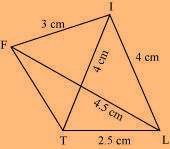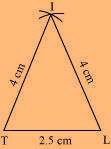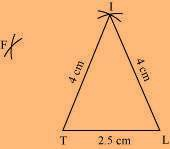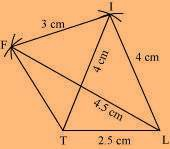# Construct the following quadrilateral: Quadrilateral LIFT. LI = 4 cm IF = 3 cm TL = 2.5 cm LF = 4.5 cm IT = 4 cm

Solution

A rough sketch of the quadrilateral LIFT can be drawn as follows.(1) ∆ ITL can be constructed by using the given measurements as follows.(2) Vertex F is 4.5 cm away from vertex L and 3 cm away from vertex I.

ConsideringL and I as centres, draw arcs of 4.5 cm radius and 3 cm radius respectively, which will be intersecting each other at point F.(3) Join F to T and F to I.